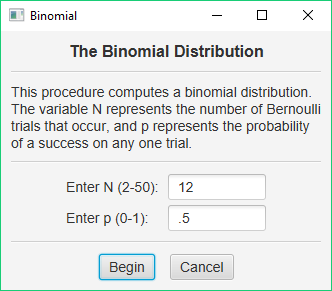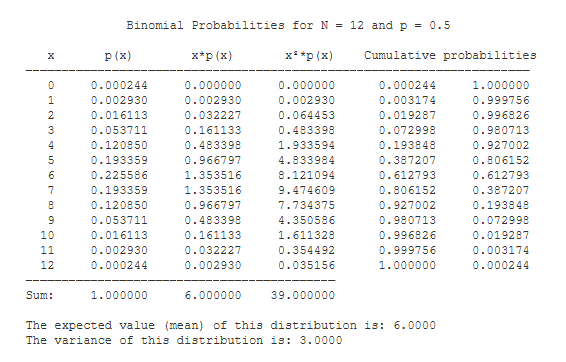# Computing a Binomial Distribution

Stats Homework includes a utility program for computing a a complete Binomial distribution with any n between 2 and 50. Pull down the Probability menu, and select Create Binomial Distribution. You will see the following dialog window:Let’s say that you would like to see a binomial distribution with N equal to 12, and with the probability of a successful outcome equal to .50. Enter these values and press the Begin button. This procedure will return the following output:The probability of a given outcome class is listed in the second column, and the cumulative probabilities for the outcome classes are listed in the last two columns. The third and fourth columns supply you with statistics that are used to compute the expected value and variance of this binomial distribution. Finally, the expected value and variance of this binomial distribution are displayed at the bottom of the output.